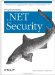# ICryptoTransform

 ICryptoTransform disposable

 System.Security.Cryptography (mscorlib.dll) interface
`public interface ICryptoTransform : IDisposable { // Public Instance Properties    public bool CanReuseTransform{get; }    public bool CanTransformMultipleBlocks{get; }    public int InputBlockSize{get; }    public int OutputBlockSize{get; } // Public Instance Methods    public int TransformBlock(byte[  ] inputBuffer, int inputOffset, int inputCount,         byte[  ] outputBuffer, int outputOffset);    public byte[  ] TransformFinalBlock(byte[  ] inputBuffer, int inputOffset, int inputCount); }`

The ICryptoTransform interface defines the basic operations of a symmetric block cipher, and is used in conjunction with the CryptoStream class to transform data. Implementations of this interface are most commonly obtained through the SymmetricAlgorithm.CreateEncryptor( ) and SymmetricAlgorithm.CreateDecryptor( ) methods.

#### Implemented By

CryptoAPITransform, FromBase64Transform, HashAlgorithm, ToBase64Transform

#### Returned By

SymmetricAlgorithm.{CreateDecryptor( ), CreateEncryptor( )}

#### Passed To

CryptoStream.CryptoStream( )Programming .Net Security
ISBN: 0596004427
EAN: 2147483647
Year: 2005
Pages: 346

Similar book on Amazon